### WEEKNUM Fx – Week Number of Date – Excel, VBA, & G Sheets

This tutorial demonstrates how to use the Excel WEEKNUM Function in Excel to get the week number in a year (1-52).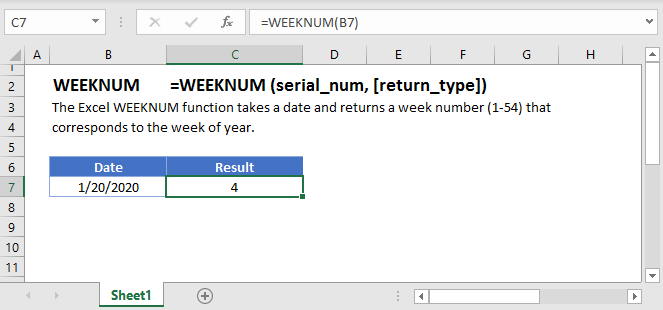## WEEKNUM Function Overview

The WEEKNUM Function Returns the week number in a year (1-52).

To use the WEEKNUM Excel Worksheet Function, Select cell and Type: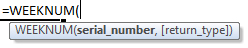(Notice how the formula input appear)

## WEEKNUM Function syntax and Inputs:

`=WEEKNUM(serial_number,return_type)`

serial_number – The date in Excel serial number format or entered as a date with quotations (“s) surround the date. Example: You can not enter 11/12/2015 directly into the cell. Instead you need to enter “11/12/2015” or you would need to use the corresponding serial number: 42320. Alternatively, you can reference a cell with the date 11/12/2015 entered. Excel automatically converts dates stored in cells into serial format (unless the date is entered as text).

return_type – OPTIONAL. A number indicating which day is the start of the week. 1 (Default) for Sunday, 2 for Monday.

AutoMacro - VBA Code Generator

## Calculate Week Number of a Date

The WEEKNUM Function calculates the week number of a date:

`=WEEKNUM(B4)`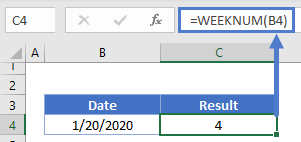The WEEKNUM function will return a value between 1 and 54. Why 54?  The function starts counting weeks on the Sunday (by default) of the week containing January 1st. This can result in 54 unique weeks in the year.

To change the weekday start, use this chart: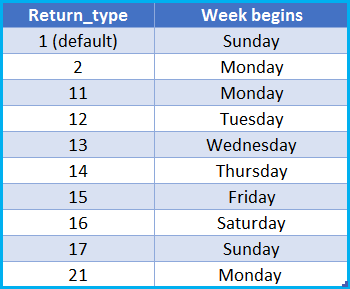This example will start the WEEKNUM function on a Monday instead:

`=WEEKNUM(B4,2)`

##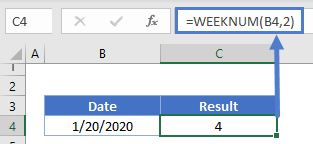WEEKNUM in Google Sheets

The WEEKNUM Function works exactly the same in Google Sheets as in Excel: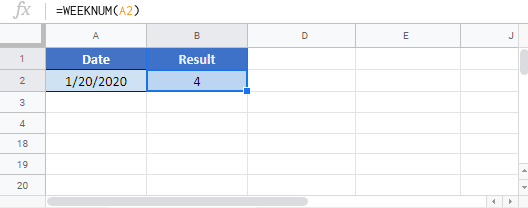## WEEKNUM Examples in VBAYou can also use the WEEKNUM function in VBA. Type:
`Application.Worksheetfunction.Weeknum(serial_number,return_type)`

We can use the WEEKNUM function as follows:

```Range("B2") = Application.WeekNum(Range("A2"))
Range("B3") = Application.WeekNum(Range("A3"))
Range("B4") = Application.WeekNum(Range("A4"))```

The result we will get is: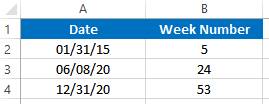For the function arguments (serial_number, etc.), you can either enter them directly into the function, or define variables to use instead.

Return to the List of all Functions in Excel

## Excel Practice Worksheet

Practice Excel functions and formulas with our 100% free practice worksheets!

• Automatically Graded Exercises
• Learn Excel, Inside Excel!